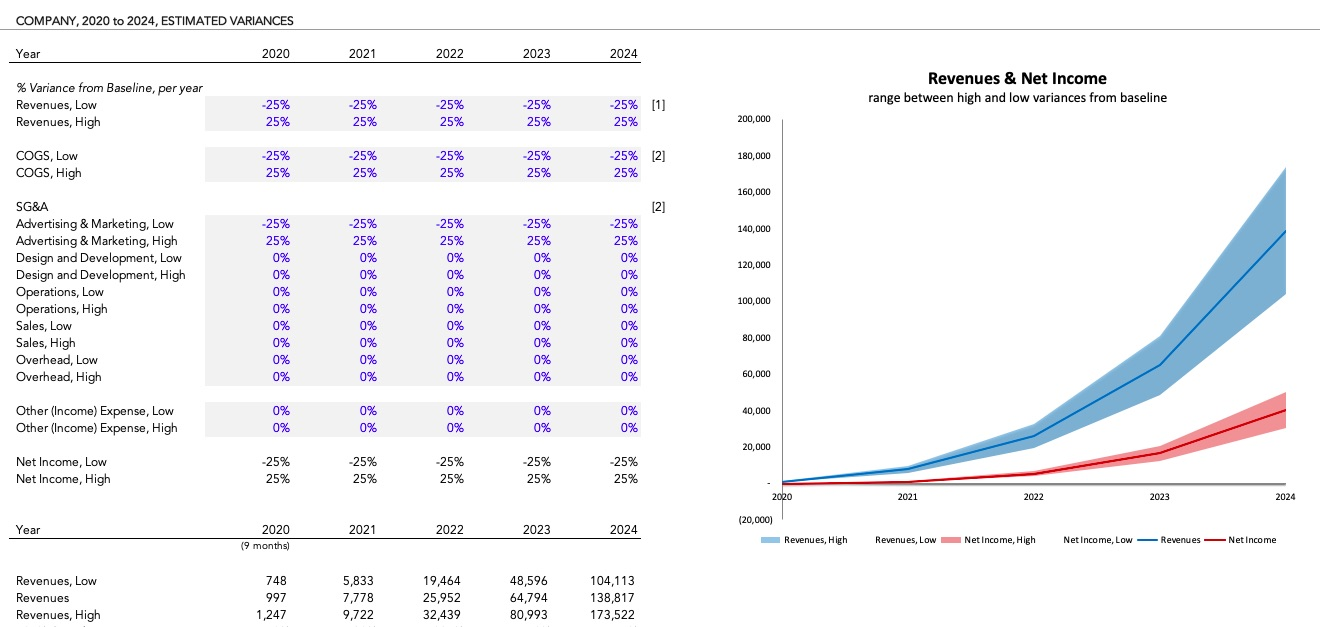# Variances

How to use the prebuilt Variances to do quick variance analysis.

Note, this sheet was deprecated in v5 of the Standard Model base.

The `Variances` sheet is an easy way to do quick analyses of ranges of revenue, cost, operating income and net income outcomes based on percentage variances from your baseline annual forecast in the model. It is not a true scenario analysis structure, as it does not change the fundamental inputs in your model or identify the root causes behind the variances, and it does not do the analysis on a monthly basis. Instead, it takes the annual results from the `Summary` and `Forecast` sheets as the baseline forecast and allows you to input the percentage variance for each high-level account (revenues, cost of sales, SG&A categories), and it calculates the resulting net income. The sheet then charts the revenues and net incomes showing the range of outcomes based on those ranges.

For true range-based analysis, use an Excel or Google Sheets add in, or check out Causal →. Here's how I use the Causal add-ins to model scenarios →

This sheet was created to help understand the impact of a decline in revenues, or changes in margins, or changes in fixed and variable costs, and see how they impact net income. The model itself is built to be used for detailed scenario analysis, with the ability to easily change an input and see the impact on the entire forecast, but sometimes that can get a bit too detailed for quick analysis and level-setting, which is why I created this sheet.

Available in the Standard Model and all Standard Model variants, v4.5.6 and above.

A couple limitations to note:

• This analysis does not recalculate cash balances at the moment
• This analysis does not create compounded changes. For example, a 25% decrease in the first year does not impact the baseline in the second year, so it does not automatically compound each year's changes. You can factor that in through your inputs, however.

## How to use #

Inputs are for the % delta from the baseline forecast. All calculations require estimates of impacts from the overall numbers, not from root input changes (growth rates, churn, average revenue, etc.). Root changes in inputs are done in the normal model inputs.## How it works #

The model uses the inputs for percentage variance from baseline (the forecast in the model, using numbers from `Summary` and `Forecast` sheets) to create two summarized profit and loss statements, showing the total impacts of all the "low" and "high" inputs. The chart then graphs the range of outcomes for revenues and net income over the five year forecast period.

## Inputs #

Inputs for this sheet are directly on the `Variances` sheet, and are input on a per-year basis.

• Terminology. The first inputs set the terms to use for the high and low variances; by default the terms are "low" and "high". You can name these whatever you want that fits your terminology, and you can switch the order (e.g. you can make the "low" label the "high" label), as long as you are consistent in your use of the variance inputs on the sheet. You can rename the labels to reflect business case scenarios - expansion, new customers, etc. - as long as your inputs reflect those labels.
• Percentage increase and decrease for each year, for each variable, which includes revenues, cost of sales, each SG&A category modeled on `Forecast`, and Other (Income) Expense. Gross Margin, EBITDA, Taxes, and Net Income are recomputed.

## Common Modifications #

None so far. This sheet can be hidden or deleted with no impact on the rest of the model.

For true range-based analysis, check out Causal.

Questions, contact me.